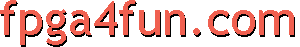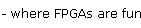## Direct Digital Synthesis 4 - Interpolation

Now, while the phase accumulator is quite precise, the output suffers from the limited number of entries in the lookup table: the output value "jumps" when going from one entry to the next. This is particularly sensible for low output frequencies, but affects high output frequencies too, and this introduces unwanted frequencies in the output spectrum.

We're going to fix that. To make it easier to understand, let's go back to a 15bit phase accumulator.

```// sine without linear interpolation
reg [14:0] phase_acc;    // 15bit
always @(posedge clk) phase_acc <= phase_acc + 15'h1;

```

The above code moves from one lookup table to the next every 16 clocks. That makes the output "jump" every 16 clocks.

An effective way to improve that is to use the lowest 4 bits of the phase accumulator (unused until now) to linearly interpolate between two successive lookup table entries. That's pretty easy to do (using two lookup tables instead of one).

```// sine with linear interpolation
reg [14:0] phase_acc;
always @(posedge clk) phase_acc <= phase_acc + 15'h1;

// use two lookup tables to get two successive table values
wire [16:0] sine1_lv, sine2_lv;

// now the 4 LSB bits from the phase accumulator need to be delayed
// (to match the latency introduced by the lookup tables)
reg [3:0] phase_LSB_delay1;  always @(posedge clk) phase_LSB_delay1 <= phase_LSB[3:0];
reg [3:0] phase_LSB_delay2;  always @(posedge clk) phase_LSB_delay2 <= phase_LSB_delay1;
reg [3:0] phase_LSB_delay3;  always @(posedge clk) phase_LSB_delay3 <= phase_LSB_delay2;

// before we can use them to do the interpolation
wire [4:0] sine1_mf = 5'h10 - phase_LSB_delay3;
wire [3:0] sine2_mf = phase_LSB_delay3;
reg [20:0] sine_p; always @(posedge clk) sine_p <= sine1_lv*sine1_mf + sine2_lv*sine2_mf;

assign DAC_data_out = sine_p[20:11];
```

Both lookup tables contain the same values. We extract a value from one, and its neighbor value from the other (the "phase_acc+1"), so that we can linearly interpolate between the two values.

The interpolation allows us to get a better resolution out of the DDS, while keeping the lookup table sizes reasonable. Our lookup tables create the sine function with 2048 values. And between each value, we interpolate 15 points, so we end-up with 2048*16=32768 sine points, much like having bigger lookup tables.

#### Ideas of improvement

• Use a 32bit phase accumulator to cover a wide range of frequencies (or check Saxo-Q's USB-controlled 32bit DDS example).
• Reduce the lookup table requirement (use only one, or using an iterative method like CORDIC).
• Use a sin(x)/x filter instead of linear interpolation.
• Use dithering to increase the DAC resolution.